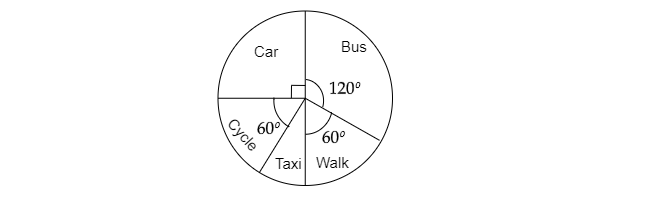"
">

# The given pie chart shows the result of a survey carried out to find the modes of travel used by children to go to school.$( a)$. What is the most common mode of transport?$( b)$. What fraction of children travel by car?$( c)$ If $18$ children travel by car, how many children took part in the survey?$( d)$ By which two modes of transport are equal number of children travelling?"

Solution:$( a)$. Since, the highest central angle $120^o$ is of the bus.

Hence, the most common mode of transport is the bus.

$( b)$.  Fraction of children travel by car$=\frac{Angle\ covered\ by\ the\ car\ section}{Total\ angle}$

$=\frac{90^o}{360^o}$

$=\frac{1}{4}$

$( c)$. No. of children travelling by car $=$ Fraction of children travelling by car $\times$ Total no of children.

$\Rightarrow 18=\frac{1}{4}\times$ Total no of children ...... from $( b)$

$\Rightarrow$ Total no of children $=18\times4$

$\Rightarrow$ Total no of children $=72$

$( d)$. The central angle for students travelling by cycle and those who walk is same. Thus , the two modes of transport are equal number of children travelling are cycle and walk.

Updated on: 10-Oct-2022

15 Views